# Mixed Effects Models - Logit Models

Jul. 2, 2021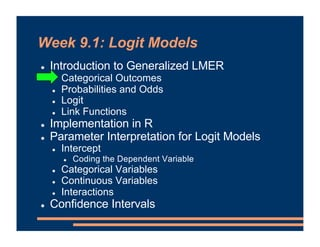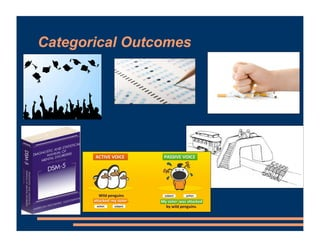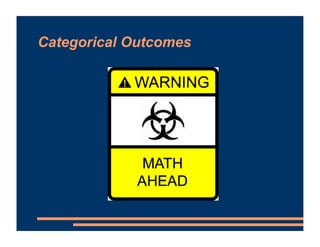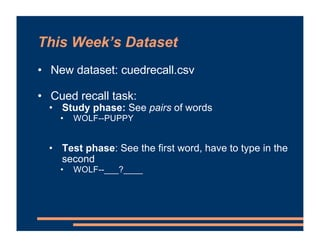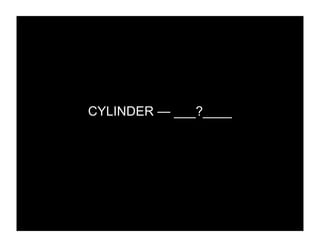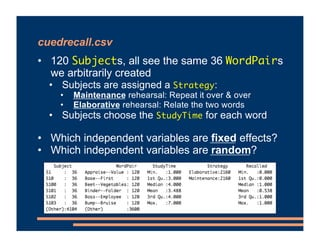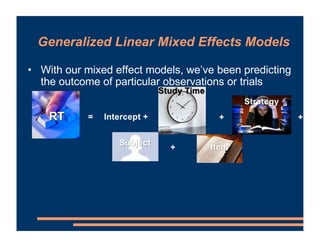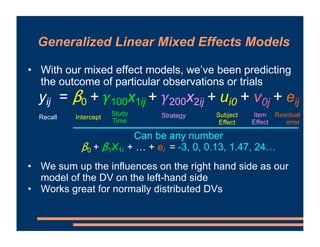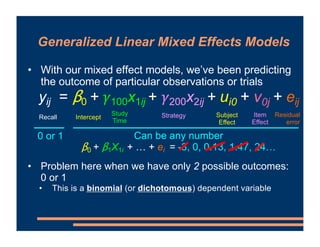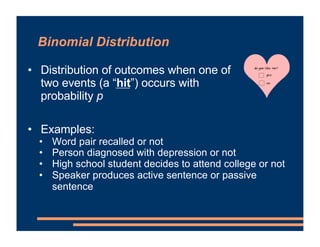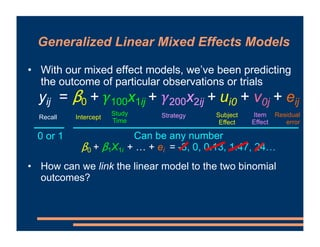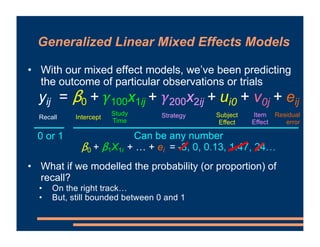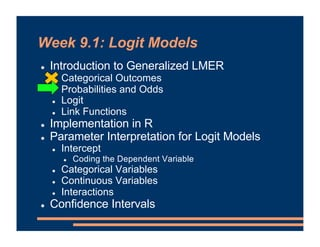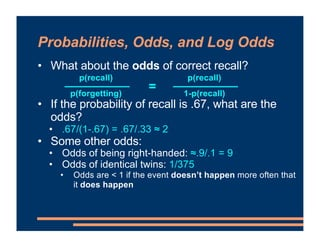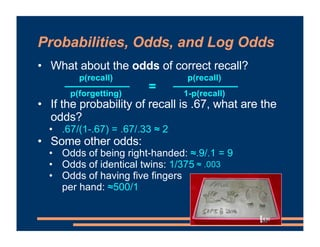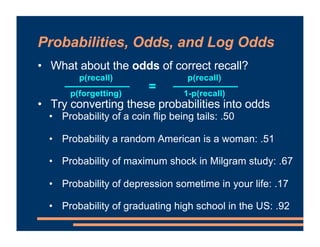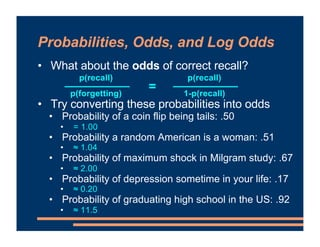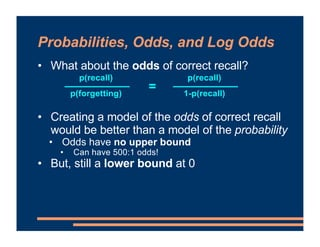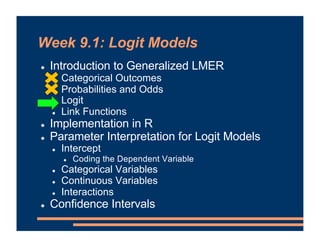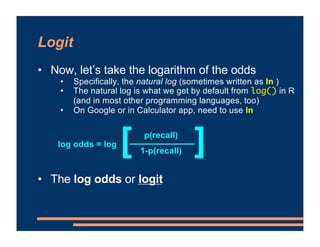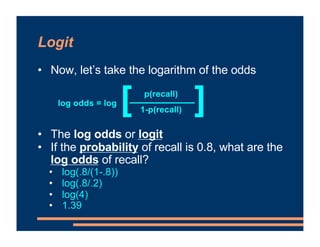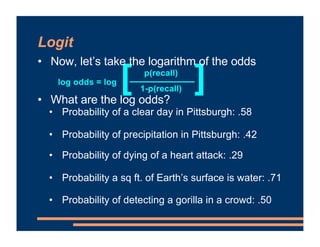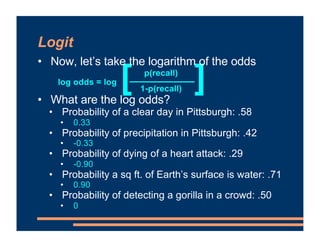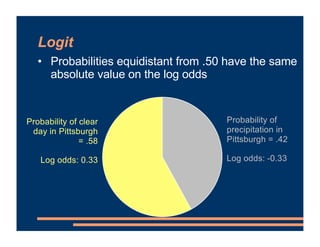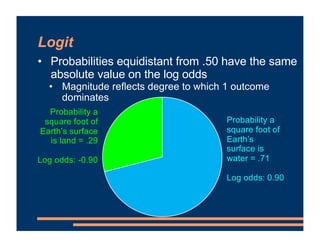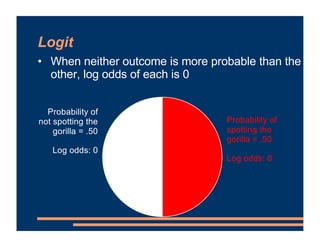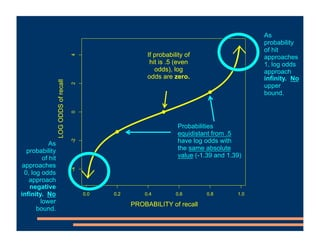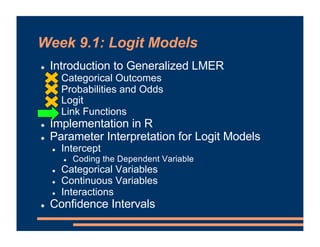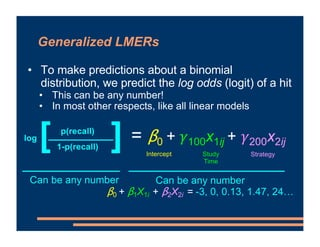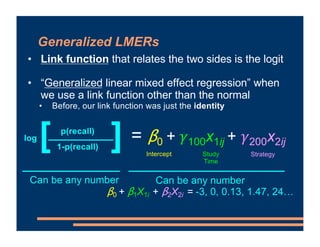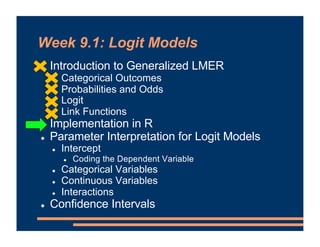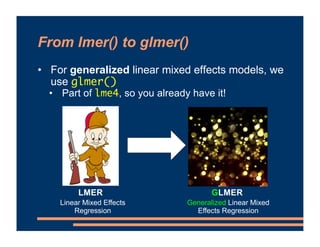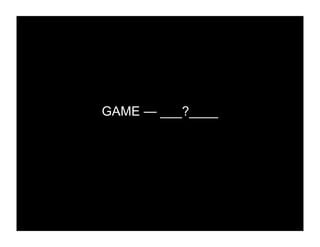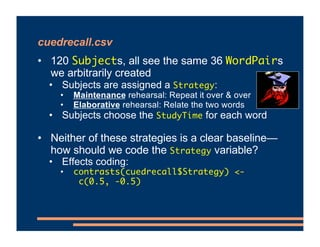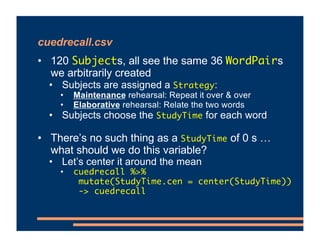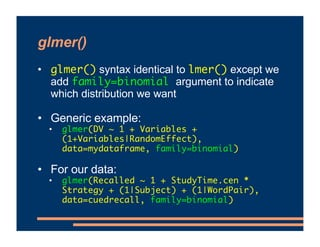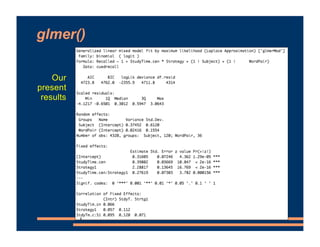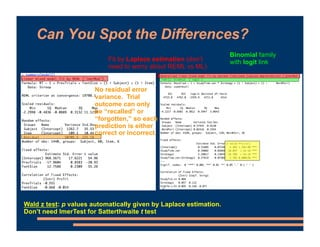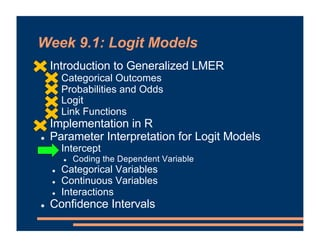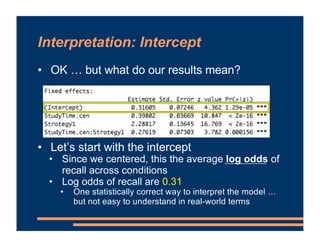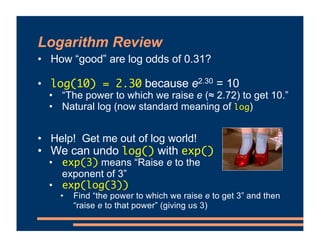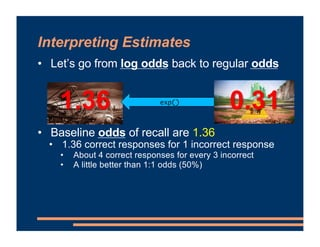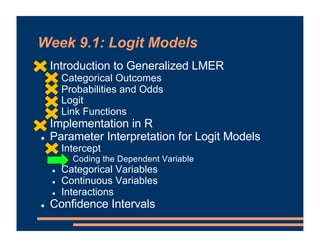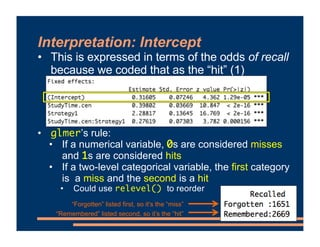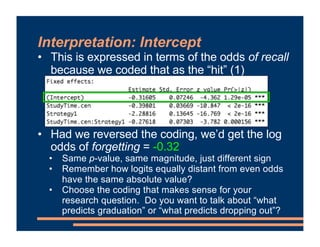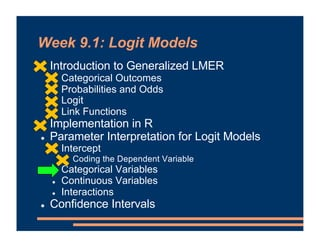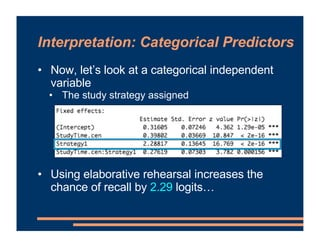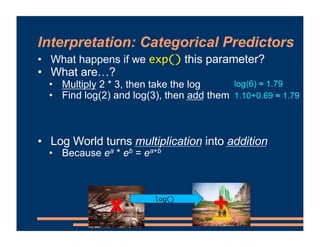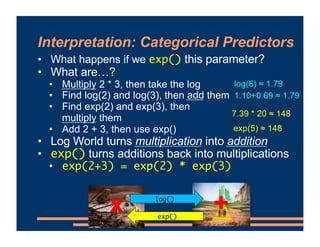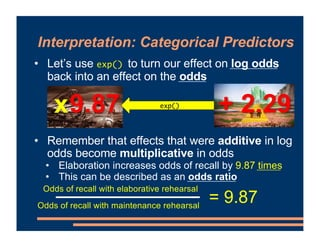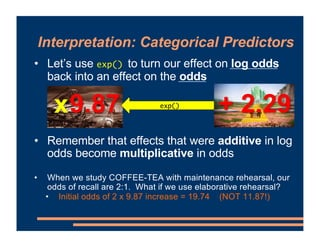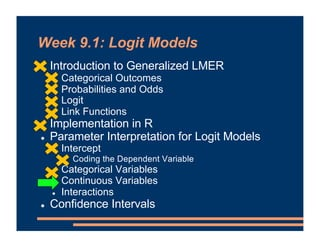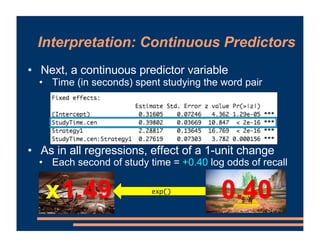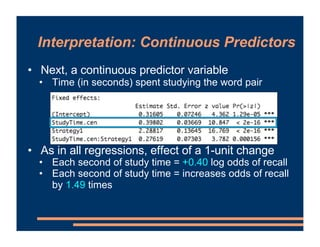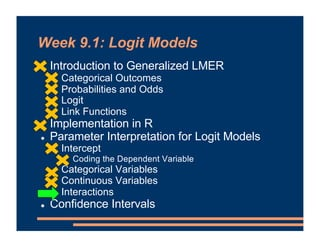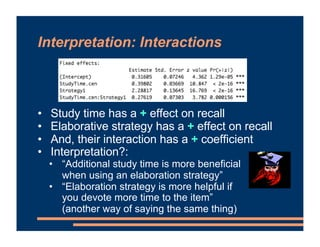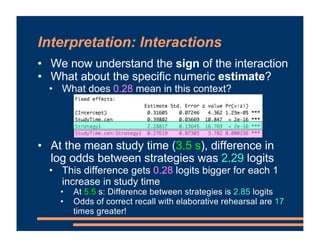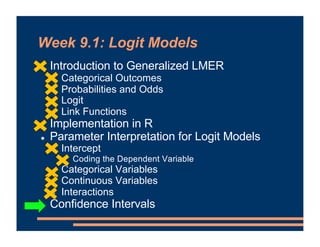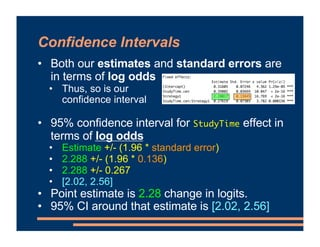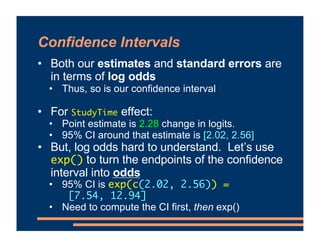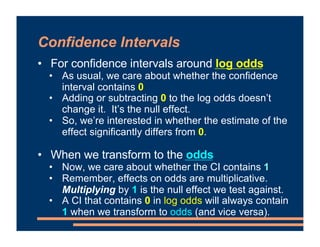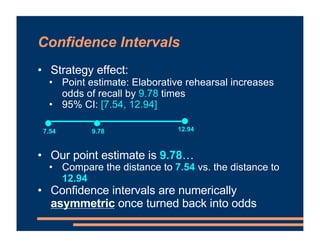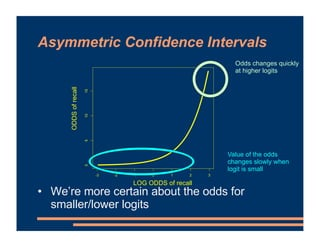1 of 71

### Mixed Effects Models - Logit Models

• 1. Week 9.1: Logit Models ! Introduction to Generalized LMER ! Categorical Outcomes ! Probabilities and Odds ! Logit ! Link Functions ! Implementation in R ! Parameter Interpretation for Logit Models ! Intercept ! Coding the Dependent Variable ! Categorical Variables ! Continuous Variables ! Interactions ! Confidence Intervals
• 2. Categorical Outcomes
• 3. Categorical Outcomes
• 4. This Week’s Dataset • New dataset: cuedrecall.csv • Cued recall task: • Study phase: See pairs of words • WOLF--PUPPY • Test phase: See the first word, have to type in the second • WOLF--___?____
• 5. CYLINDER—CAN
• 6. CAREER—JOB
• 7. EXPERT—PROFESSOR
• 8. VIKING—COLLEGE
• 9. GAME—MONOPOLY
• 11. CYLINDER — ___?____
• 12. EXPERT — ___?____
• 13. cuedrecall.csv • 120 Subjects, all see the same 36 WordPairs we arbitrarily created • Subjects are assigned a Strategy: • Maintenance rehearsal: Repeat it over & over • Elaborative rehearsal: Relate the two words • Subjects choose the StudyTime for each word • Which independent variables are fixed effects? • Which independent variables are random?
• 14. • With our mixed effect models, we’ve been predicting the outcome of particular observations or trials Generalized Linear Mixed Effects Models = Intercept + + Study Time Subject + Item RT + Strategy
• 15. • With our mixed effect models, we’ve been predicting the outcome of particular observations or trials • We sum up the influences on the right hand side as our model of the DV on the left-hand side • Works great for normally distributed DVs Generalized Linear Mixed Effects Models yij = β0 + !100x1ij + !200x2ij + ui0 + v0j + eij Intercept Study Time Strategy Residual error Subject Effect Item Effect Can be any number β0 + β1X1i + … + ei = -3, 0, 0.13, 1.47, 24… Recall
• 16. • With our mixed effect models, we’ve been predicting the outcome of particular observations or trials • Problem here when we have only 2 possible outcomes: 0 or 1 • This is a binomial (or dichotomous) dependent variable Generalized Linear Mixed Effects Models yij = β0 + !100x1ij + !200x2ij + ui0 + v0j + eij Intercept Study Time Strategy Residual error Subject Effect Item Effect 0 or 1 Can be any number β0 + β1X1i + … + ei = -3, 0, 0.13, 1.47, 24… Recall
• 17. Binomial Distribution • Distribution of outcomes when one of two events (a “hit”) occurs with probability p • Examples: • Word pair recalled or not • Person diagnosed with depression or not • High school student decides to attend college or not • Speaker produces active sentence or passive sentence
• 18. • With our mixed effect models, we’ve been predicting the outcome of particular observations or trials • How can we link the linear model to the two binomial outcomes? Generalized Linear Mixed Effects Models yij = β0 + !100x1ij + !200x2ij + ui0 + v0j + eij Intercept Study Time Strategy Residual error Subject Effect Item Effect 0 or 1 Can be any number β0 + β1X1i + … + ei = -3, 0, 0.13, 1.47, 24… Recall
• 19. • With our mixed effect models, we’ve been predicting the outcome of particular observations or trials • What if we modelled the probability (or proportion) of recall? • On the right track… • But, still bounded between 0 and 1 Generalized Linear Mixed Effects Models yij = β0 + !100x1ij + !200x2ij + ui0 + v0j + eij Intercept Study Time Strategy Residual error Subject Effect Item Effect 0 or 1 Can be any number β0 + β1X1i + … + ei = -3, 0, 0.13, 1.47, 24… Recall
• 20. Week 9.1: Logit Models ! Introduction to Generalized LMER ! Categorical Outcomes ! Probabilities and Odds ! Logit ! Link Functions ! Implementation in R ! Parameter Interpretation for Logit Models ! Intercept ! Coding the Dependent Variable ! Categorical Variables ! Continuous Variables ! Interactions ! Confidence Intervals
• 21. Probabilities, Odds, and Log Odds • What about the odds of correct recall? • If the probability of recall is .67, what are the odds? • .67/(1-.67) = .67/.33 ≈ 2 • Some other odds: • Odds of being right-handed: ≈.9/.1 = 9 • Odds of identical twins: 1/375 • Odds are < 1 if the event doesn’t happen more often that it does happen p(recall) p(recall) 1-p(recall) = p(forgetting)
• 22. Probabilities, Odds, and Log Odds • What about the odds of correct recall? • If the probability of recall is .67, what are the odds? • .67/(1-.67) = .67/.33 ≈ 2 • Some other odds: • Odds of being right-handed: ≈.9/.1 = 9 • Odds of identical twins: 1/375 • Odds of having five fingers per hand: ≈500/1 p(recall) p(recall) 1-p(recall) = ≈ .003 p(forgetting)
• 23. Probabilities, Odds, and Log Odds • What about the odds of correct recall? • Try converting these probabilities into odds • Probability of a coin flip being tails: .50 • Probability a random American is a woman: .51 • Probability of maximum shock in Milgram study: .67 • Probability of depression sometime in your life: .17 • Probability of graduating high school in the US: .92 p(recall) p(forgetting) p(recall) 1-p(recall) =
• 24. • What about the odds of correct recall? • Try converting these probabilities into odds • Probability of a coin flip being tails: .50 • = 1.00 • Probability a random American is a woman: .51 • ≈ 1.04 • Probability of maximum shock in Milgram study: .67 • ≈ 2.00 • Probability of depression sometime in your life: .17 • ≈ 0.20 • Probability of graduating high school in the US: .92 • ≈ 11.5 Probabilities, Odds, and Log Odds p(recall) p(forgetting) p(recall) 1-p(recall) =
• 25. Probabilities, Odds, and Log Odds • What about the odds of correct recall? • Creating a model of the odds of correct recall would be better than a model of the probability • Odds have no upper bound • Can have 500:1 odds! • But, still a lower bound at 0 p(recall) p(forgetting) p(recall) 1-p(recall) =
• 26. Week 9.1: Logit Models ! Introduction to Generalized LMER ! Categorical Outcomes ! Probabilities and Odds ! Logit ! Link Functions ! Implementation in R ! Parameter Interpretation for Logit Models ! Intercept ! Coding the Dependent Variable ! Categorical Variables ! Continuous Variables ! Interactions ! Confidence Intervals
• 27. Logit • Now, let’s take the logarithm of the odds • Specifically, the natural log (sometimes written as ln ) • The natural log is what we get by default from log() in R (and in most other programming languages, too) • On Google or in Calculator app, need to use ln • The log odds or logit p(recall) 1-p(recall) [ ] log odds = log
• 28. Logit • Now, let’s take the logarithm of the odds • The log odds or logit • If the probability of recall is 0.8, what are the log odds of recall? • log(.8/(1-.8)) • log(.8/.2) • log(4) • 1.39 p(recall) 1-p(recall) [ ] log odds = log
• 29. [ ] Logit • Now, let’s take the logarithm of the odds • What are the log odds? • Probability of a clear day in Pittsburgh: .58 • Probability of precipitation in Pittsburgh: .42 • Probability of dying of a heart attack: .29 • Probability a sq ft. of Earth’s surface is water: .71 • Probability of detecting a gorilla in a crowd: .50 p(recall) 1-p(recall) log odds = log
• 30. [ ] Logit • Now, let’s take the logarithm of the odds • What are the log odds? • Probability of a clear day in Pittsburgh: .58 • 0.33 • Probability of precipitation in Pittsburgh: .42 • -0.33 • Probability of dying of a heart attack: .29 • -0.90 • Probability a sq ft. of Earth’s surface is water: .71 • 0.90 • Probability of detecting a gorilla in a crowd: .50 • 0 p(recall) 1-p(recall) log odds = log
• 31. Logit • Probabilities equidistant from .50 have the same absolute value on the log odds Probability of precipitation in Pittsburgh = .42 Log odds: -0.33 Probability of clear day in Pittsburgh = .58 Log odds: 0.33
• 32. Logit • Probabilities equidistant from .50 have the same absolute value on the log odds • Magnitude reflects degree to which 1 outcome dominates Probability a square foot of Earth’s surface is water = .71 Log odds: 0.90 Probability a square foot of Earth’s surface is land = .29 Log odds: -0.90
• 33. Logit • When neither outcome is more probable than the other, log odds of each is 0 Probability of spotting the gorilla = .50 Log odds: 0 Probability of not spotting the gorilla = .50 Log odds: 0
• 34. 0.0 0.2 0.4 0.6 0.8 1.0 -4 -2 0 2 4 PROBABILITY of recall LOG ODDS of recall As probability of hit approaches 1, log odds approach infinity. No upper bound. As probability of hit approaches 0, log odds approach negative infinity. No lower bound. If probability of hit is .5 (even odds), log odds are zero. Probabilities equidistant from .5 have log odds with the same absolute value (-1.39 and 1.39) PROBABILITY of recall LOG ODDS of recall
• 35. Week 9.1: Logit Models ! Introduction to Generalized LMER ! Categorical Outcomes ! Probabilities and Odds ! Logit ! Link Functions ! Implementation in R ! Parameter Interpretation for Logit Models ! Intercept ! Coding the Dependent Variable ! Categorical Variables ! Continuous Variables ! Interactions ! Confidence Intervals
• 36. Generalized LMERs • To make predictions about a binomial distribution, we predict the log odds (logit) of a hit • This can be any number! • In most other respects, like all linear models = β0 + !100x1ij + !200x2ij Intercept Study Time Strategy Can be any number p(recall) 1-p(recall) [ ] log Can be any number β0 + β1X1i + β2X2i = -3, 0, 0.13, 1.47, 24…
• 37. Generalized LMERs • Link function that relates the two sides is the logit • “Generalized linear mixed effect regression” when we use a link function other than the normal • Before, our link function was just the identity = β0 + !100x1ij + !200x2ij Intercept Study Time Strategy Can be any number p(recall) 1-p(recall) [ ] log Can be any number β0 + β1X1i + β2X2i = -3, 0, 0.13, 1.47, 24…
• 38. Week 9.1: Logit Models ! Introduction to Generalized LMER ! Categorical Outcomes ! Probabilities and Odds ! Logit ! Link Functions ! Implementation in R ! Parameter Interpretation for Logit Models ! Intercept ! Coding the Dependent Variable ! Categorical Variables ! Continuous Variables ! Interactions ! Confidence Intervals
• 39. From lmer() to glmer() • For generalized linear mixed effects models, we use glmer() • Part of lme4, so you already have it! LMER Linear Mixed Effects Regression GLMER Generalized Linear Mixed Effects Regression
• 40. VIKING — ___?____
• 41. GAME — ___?____
• 42. cuedrecall.csv • 120 Subjects, all see the same 36 WordPairs we arbitrarily created • Subjects are assigned a Strategy: • Maintenance rehearsal: Repeat it over & over • Elaborative rehearsal: Relate the two words • Subjects choose the StudyTime for each word • Neither of these strategies is a clear baseline— how should we code the Strategy variable? • Effects coding: • contrasts(cuedrecall\$Strategy) <- c(0.5, -0.5)
• 43. cuedrecall.csv • 120 Subjects, all see the same 36 WordPairs we arbitrarily created • Subjects are assigned a Strategy: • Maintenance rehearsal: Repeat it over & over • Elaborative rehearsal: Relate the two words • Subjects choose the StudyTime for each word • There’s no such thing as a StudyTime of 0 s … what should we do this variable? • Let’s center it around the mean • cuedrecall %>% mutate(StudyTime.cen = center(StudyTime)) -> cuedrecall
• 44. glmer() • glmer() syntax identical to lmer() except we add family=binomial argument to indicate which distribution we want • Generic example: • glmer(DV ~ 1 + Variables + (1+Variables|RandomEffect), data=mydataframe, family=binomial) • For our data: • glmer(Recalled ~ 1 + StudyTime.cen * Strategy + (1|Subject) + (1|WordPair), data=cuedrecall, family=binomial)
• 45. glmer() Our present results
• 46. Can You Spot the Differences? Binomial family with logit link Fit by Laplace estimation (don’t need to worry about REML vs ML) Wald z test: p values automatically given by Laplace estimation. Don’t need lmerTest for Satterthwaite t test No residual error variance. Trial outcome can only be “recalled” or “forgotten,” so each prediction is either correct or incorrect.
• 47. Week 9.1: Logit Models ! Introduction to Generalized LMER ! Categorical Outcomes ! Probabilities and Odds ! Logit ! Link Functions ! Implementation in R ! Parameter Interpretation for Logit Models ! Intercept ! Coding the Dependent Variable ! Categorical Variables ! Continuous Variables ! Interactions ! Confidence Intervals
• 48. Interpretation: Intercept • OK … but what do our results mean? • Let’s start with the intercept • Since we centered, this the average log odds of recall across conditions • Log odds of recall are 0.31 • One statistically correct way to interpret the model … but not easy to understand in real-world terms
• 49. Logarithm Review • How “good” are log odds of 0.31? • log(10) = 2.30 because e2.30 = 10 • “The power to which we raise e (≈ 2.72) to get 10.” • Natural log (now standard meaning of log) • Help! Get me out of log world! • We can undo log() with exp() • exp(3) means “Raise e to the exponent of 3” • exp(log(3)) • Find “the power to which we raise e to get 3” and then “raise e to that power” (giving us 3)
• 50. Interpreting Estimates • Let’s go from log odds back to regular odds • Baseline odds of recall are 1.36 • 1.36 correct responses for 1 incorrect response • About 4 correct responses for every 3 incorrect • A little better than 1:1 odds (50%) 1.36 0.31 exp()
• 51. Week 9.1: Logit Models ! Introduction to Generalized LMER ! Categorical Outcomes ! Probabilities and Odds ! Logit ! Link Functions ! Implementation in R ! Parameter Interpretation for Logit Models ! Intercept ! Coding the Dependent Variable ! Categorical Variables ! Continuous Variables ! Interactions ! Confidence Intervals
• 52. Interpretation: Intercept • This is expressed in terms of the odds of recall because we coded that as the “hit” (1) • glmer’s rule: • If a numerical variable, 0s are considered misses and 1s are considered hits • If a two-level categorical variable, the first category is a miss and the second is a hit • Could use relevel() to reorder “Forgotten” listed first, so it’s the “miss” “Remembered” listed second, so it’s the “hit”
• 53. Interpretation: Intercept • This is expressed in terms of the odds of recall because we coded that as the “hit” (1) • Had we reversed the coding, we’d get the log odds of forgetting = -0.32 • Same p-value, same magnitude, just different sign • Remember how logits equally distant from even odds have the same absolute value? • Choose the coding that makes sense for your research question. Do you want to talk about “what predicts graduation” or “what predicts dropping out”?
• 54. Week 9.1: Logit Models ! Introduction to Generalized LMER ! Categorical Outcomes ! Probabilities and Odds ! Logit ! Link Functions ! Implementation in R ! Parameter Interpretation for Logit Models ! Intercept ! Coding the Dependent Variable ! Categorical Variables ! Continuous Variables ! Interactions ! Confidence Intervals
• 55. Interpretation: Categorical Predictors • Now, let’s look at a categorical independent variable • The study strategy assigned • Using elaborative rehearsal increases the chance of recall by 2.29 logits…
• 56. Interpretation: Categorical Predictors • What happens if we exp() this parameter? • What are…? • Multiply 2 * 3, then take the log • Find log(2) and log(3), then add them • Log World turns multiplication into addition • Because ea * eb = ea+b x + log() log(6) ≈ 1.79 1.10+0.69 ≈ 1.79
• 57. Interpretation: Categorical Predictors • What happens if we exp() this parameter? • What are…? • Multiply 2 * 3, then take the log • Find log(2) and log(3), then add them • Find exp(2) and exp(3), then multiply them • Add 2 + 3, then use exp() • Log World turns multiplication into addition • exp() turns additions back into multiplications • exp(2+3) = exp(2) * exp(3) x + log() exp() log(6) ≈ 1.79 1.10+0.69 ≈ 1.79 7.39 * 20 ≈ 148 exp(5) ≈ 148
• 58. Interpretation: Categorical Predictors • Let’s use exp() to turn our effect on log odds back into an effect on the odds • Remember that effects that were additive in log odds become multiplicative in odds • Elaboration increases odds of recall by 9.87 times • This can be described as an odds ratio 9.87 + 2.29 exp() x Odds of recall with elaborative rehearsal Odds of recall with maintenance rehearsal = 9.87
• 59. Interpretation: Categorical Predictors • Let’s use exp() to turn our effect on log odds back into an effect on the odds • Remember that effects that were additive in log odds become multiplicative in odds • When we study COFFEE-TEA with maintenance rehearsal, our odds of recall are 2:1. What if we use elaborative rehearsal? • Initial odds of 2 x 9.87 increase = 19.74 (NOT 11.87!) 9.87 + 2.29 exp() x
• 60. Week 9.1: Logit Models ! Introduction to Generalized LMER ! Categorical Outcomes ! Probabilities and Odds ! Logit ! Link Functions ! Implementation in R ! Parameter Interpretation for Logit Models ! Intercept ! Coding the Dependent Variable ! Categorical Variables ! Continuous Variables ! Interactions ! Confidence Intervals
• 61. Interpretation: Continuous Predictors • Next, a continuous predictor variable • Time (in seconds) spent studying the word pair • As in all regressions, effect of a 1-unit change • Each second of study time = +0.40 log odds of recall 1.49 0.40 exp() x
• 62. Interpretation: Continuous Predictors • Next, a continuous predictor variable • Time (in seconds) spent studying the word pair • As in all regressions, effect of a 1-unit change • Each second of study time = +0.40 log odds of recall • Each second of study time = increases odds of recall by 1.49 times
• 63. Week 9.1: Logit Models ! Introduction to Generalized LMER ! Categorical Outcomes ! Probabilities and Odds ! Logit ! Link Functions ! Implementation in R ! Parameter Interpretation for Logit Models ! Intercept ! Coding the Dependent Variable ! Categorical Variables ! Continuous Variables ! Interactions ! Confidence Intervals
• 64. Interpretation: Interactions • Study time has a + effect on recall • Elaborative strategy has a + effect on recall • And, their interaction has a + coefficient • Interpretation?: • “Additional study time is more beneficial when using an elaboration strategy” • “Elaboration strategy is more helpful if you devote more time to the item” (another way of saying the same thing)
• 65. Interpretation: Interactions • We now understand the sign of the interaction • What about the specific numeric estimate? • What does 0.28 mean in this context? • At the mean study time (3.5 s), difference in log odds between strategies was 2.29 logits • This difference gets 0.28 logits bigger for each 1 increase in study time • At 5.5 s: Difference between strategies is 2.85 logits • Odds of correct recall with elaborative rehearsal are 17 times greater!
• 66. Week 9.1: Logit Models ! Introduction to Generalized LMER ! Categorical Outcomes ! Probabilities and Odds ! Logit ! Link Functions ! Implementation in R ! Parameter Interpretation for Logit Models ! Intercept ! Coding the Dependent Variable ! Categorical Variables ! Continuous Variables ! Interactions ! Confidence Intervals
• 67. Confidence Intervals • Both our estimates and standard errors are in terms of log odds • Thus, so is our confidence interval • 95% confidence interval for StudyTime effect in terms of log odds • Estimate +/- (1.96 * standard error) • 2.288 +/- (1.96 * 0.136) • 2.288 +/- 0.267 • [2.02, 2.56] • Point estimate is 2.28 change in logits. • 95% CI around that estimate is [2.02, 2.56]
• 68. Confidence Intervals • Both our estimates and standard errors are in terms of log odds • Thus, so is our confidence interval • For StudyTime effect: • Point estimate is 2.28 change in logits. • 95% CI around that estimate is [2.02, 2.56] • But, log odds hard to understand. Let’s use exp() to turn the endpoints of the confidence interval into odds • 95% CI is exp(c(2.02, 2.56)) = [7.54, 12.94] • Need to compute the CI first, then exp()
• 69. Confidence Intervals • For confidence intervals around log odds • As usual, we care about whether the confidence interval contains 0 • Adding or subtracting 0 to the log odds doesn’t change it. It’s the null effect. • So, we’re interested in whether the estimate of the effect significantly differs from 0. • When we transform to the odds • Now, we care about whether the CI contains 1 • Remember, effects on odds are multiplicative. Multiplying by 1 is the null effect we test against. • A CI that contains 0 in log odds will always contain 1 when we transform to odds (and vice versa).
• 70. Confidence Intervals • Strategy effect: • Point estimate: Elaborative rehearsal increases odds of recall by 9.78 times • 95% CI: [7.54, 12.94] • Our point estimate is 9.78… • Compare the distance to 7.54 vs. the distance to 12.94 • Confidence intervals are numerically asymmetric once turned back into odds 9.78 7.54 12.94
• 71. -3 -2 -1 0 1 2 3 0 5 10 15 LOG ODDS of recall ODDS of recall Value of the odds changes slowly when logit is small LOG ODDS of recall Odds changes quickly at higher logits ODDS of recall Asymmetric Confidence Intervals • We’re more certain about the odds for smaller/lower logits